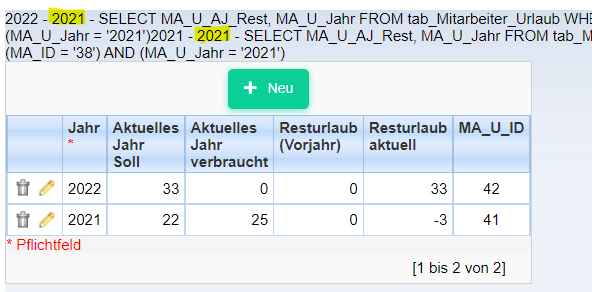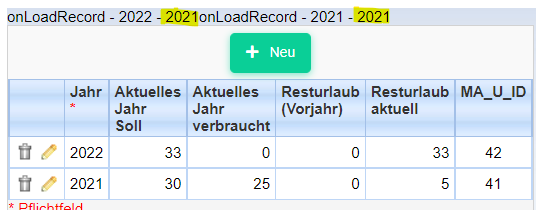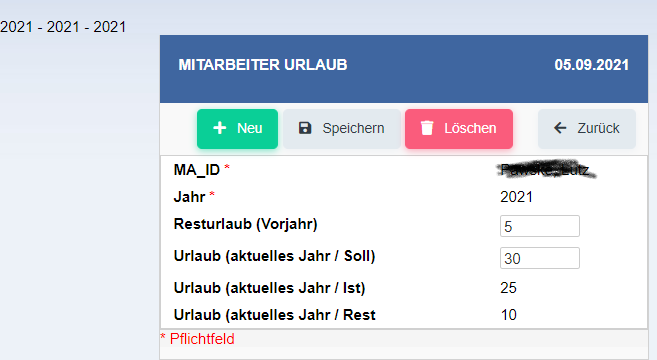Hi,

I have a small problem with my PHP code and I need a little help, please.

In the onLoadRecord event I defined a SQL call to get values from an other record (previous year) based on the year (\$v_AJ) of the current record

\$v_AJ = {MA_U_Jahr};
\$v_VJ = 0;
\$v_VJ = date(“Y”, strtotime(\$v_AJ - 1));

\$check_sql = “SELECT MA_U_AJ_Rest, MA_U_Jahr”
. " FROM tab_Mitarbeiter_Urlaub"
. " WHERE (MA_ID = ‘" . {MA_ID} . "’) AND (MA_U_Jahr = ‘" . \$v_VJ . "’)";

sc_lookup(rs, \$check_sql);

if (isset({rs})) // Row found
{
\$v_UR_VJ_R = {rs};
}
else // No row found
{
\$v_UR_VJ_R = 0;
}

echo \$v_AJ . " - " . \$v_VJ . " - " . \$check_sql;

The first record works fine, but the second recors use the same value from the variable \$v_VJWhat did I wrong ?

Mmmm.

echo \$v_AJ . " - " . \$v_VJ ;

just after

\$v_VJ = date(“Y”, strtotime(\$v_AJ - 1));

and comment out all the SQL block

What is the value displayed ?

Hi maxi

here the example with a form as editable grid view

\$v_AJ = {MA_U_Jahr};
\$v_VJ = date(“Y”, strtotime(\$v_AJ - 1));

echo "onLoadRecord - " . \$v_AJ . " - " . \$v_VJ;and here an example with a single form

\$v_AJ = {MA_U_Jahr};
\$v_VJ = date(“Y”, strtotime({MA_U_Jahr} - 1));
echo {MA_U_Jahr} . " - " . \$v_AJ . " - " . \$v_VJ;Hy ,
date function return the current time (in your case the current year 2021) if the second argument is not a proper timestamp.

Hi Maxi,
the problem for the single form issue was sitting at the keyboard.
The field {MA_U_Jahr} in an integer fiel which contain the year value.
So a conversion with the date function is not nessecary.

Hi maxi,